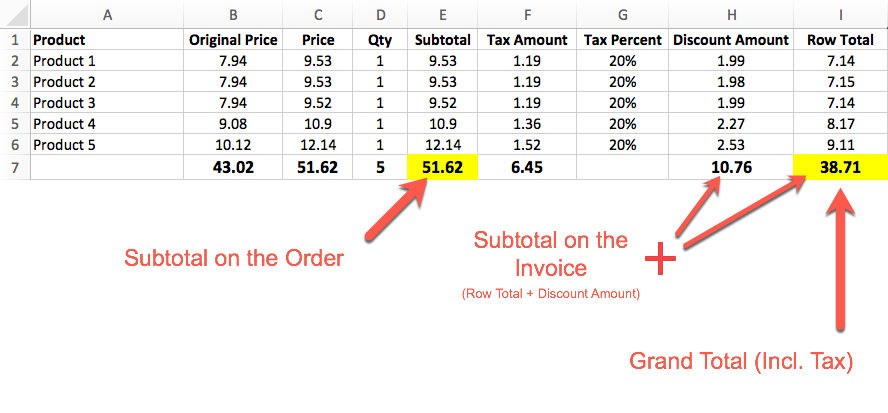# How Order and Invoice Totals are Calculated

Decrypting the Totals and Price columns of your Order and Invoice

### 🚧How Totals are calculated depends on your Tax Settings

In Setup>Tax>Tax Settings there are several configurations that can change how the totals are calculated and/or displayed. This Article gives a basic overview of the possible permutations.

# Sample Order

This sample order actually shows how the Subtotal on the Order can be different from the Subtotal on the Invoice:In this example above, you will actually see that the Subtotal Column minus the Discount Amount Column does NOT equal the Row Total column! This is correct because based on this user's settings, the Row Total = Original Price Minus Discount Amount, Plus Tax Amount.

# Column Calculations

Note: Calculations may vary based on settings in Setup>Tax>Tax Settings

Column

Calculation

Original Price

Price of the product (respects Incl/Excl Tax Setting)

Price

Original Price Plus Tax Percent (if Original Price is Excl. Tax)

Subtotal

Price * Qty

Tax Amount

Tax Percent * Original Price (if Excl. Tax) subtracted by Discount Amount
(Order of operations depends on Tax Settings for Discounts)

Row Total

Original Price Minus Discount Amount, Plus Tax Amount.
(Order of operations depends on Tax Settings for Discounts)

# Totals Calculations

Note: Calculations may vary based on settings in Setup>Tax>Tax Settings

Total

Calculation

Order Subtotal

Sum of Subtotal Column

Invoice Subtotal

Sum of Row Total + Discount Amount

Grand Total

Sum of Row Total Column

# Examples

## Applying Tax After Discount:

Base Price

Discount 20%

Price after discount

Tax (15%)

Price + Tax = Row Total

\$15

\$3

\$12

\$1.80

\$13.80